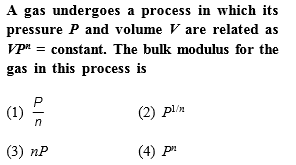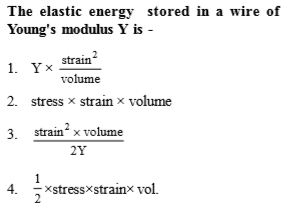[Only for Dropper and XII batch]

Complete Question Bank + Test Series
Complete Question Bank

Difficulty Level:Complete Question Bank + Test Series
Complete Question Bank

Difficulty Level:

The bulk modulus of a spherical object is B. If it is subjected to uniform pressure p, the fractional decrease in radius is

(a)$\frac{P}{B}$

(b) $\frac{B}{3p}$

(c) $\frac{3p}{B}$

(d)$\frac{p}{3B}$

Complete Question Bank + Test Series
Complete Question Bank

Difficulty Level:

The Young's modulus of steel is twice that of brass. Two wires of same length and of same area of cross-section, one of steel and another of brass are suspended from the same roof. If we want the lower ends of the wires to be at the same level, then the weight added to the steel and brass wires must be in the ratio of

(a)1:2

(b)2:1

(c)4:1

(d)1:1

Complete Question Bank + Test Series
Complete Question Bank

Difficulty Level:

The Young's modulus of a wire of length L and radius r is If the length and radius are reduced to L/2 and r/2, then its Young's modulus will be -

(a) 1:1                           (b) 1:4

(c) 1:8                             (d) 8:1

Complete Question Bank + Test Series
Complete Question Bank

Difficulty Level:

A force F is needed to break a copper wire having radius R. The force needed to break a copper wire of radius 2R will be

(a) F/2                                      (b) 2F

(c) 4F                                       (d) F/4

Complete Question Bank + Test Series
Complete Question Bank

Difficulty Level:

The relationship between Young's modulus Y, Bulk modulus K and modulus of rigidity n is

(a) $Y=\frac{9nK}{n+3K}$                                    (b) $\frac{9YK}{Y+3K}$

(c)  $Y=\frac{9nK}{3+K}$                                     (d) $Y=\frac{3nK}{9n+K}$

Complete Question Bank + Test Series
Complete Question Bank

Difficulty Level:

If x longitudinal strain is produced in a wire of Young's modulus y, then energy stored in the material of the wire per unit volume is-

(a) $y{x}^{2}$                                 (b) $2y{x}^{2}$

(c) $\frac{1}{2}{y}^{2}x$                             (d) $\frac{1}{2}y{x}^{2}$

Complete Question Bank + Test Series
Complete Question Bank

Difficulty Level:

The Young's modulus of a rubber string 8 cm long and density  is , is suspended on the ceiling in a room. The increase in length due to its own weight will be

(a)                            (b) $9.6×{10}^{-11}m$

(c)                            (d) 9.6 m

Complete Question Bank + Test Series
Complete Question Bank

Difficulty Level:

A and B are two wires of same material. The radius of A is twice that of B. They are stretched by the same load. Then the stress on B is

(a) Equal to that on A                  (b) Four times that on A

(c) Two times that on A               (d) Half that on A